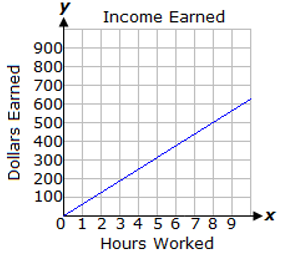Mathematics
Easy

Question

# Write statement below accurately represents the graph.## \$100 is earned for each hour worked\$200 earned for every 2 hours worked is the unit rate\$500 is earned for every 8 hours worked\$300 is earned after working 4 hoursHint:

## The correct answer is: \$500 is earned for every 8 hours worked

### Here, a graph showing the amount earned per hour is given.We have to find which of the given options is correct.According to the given graph, \$500 is earned for 8 hour of work.Hence, the correct option is B.

The graph of a direct variation is a straight line.#### With Turito Foundation.#### Get an Expert Advice From Turito.